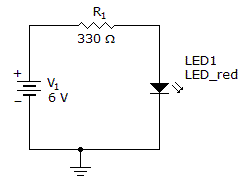# Electronics - Diodes and Applications

### Exercise :: Diodes and Applications - General Questions

6.

When matching polarity connections have been made and the potential difference (PD) is above 0.7 V, the diode is considered to be:

 A. not working B. forward biased C. reverse biased D. an open switch

Answer: Option B

Explanation:

No answer description available for this question. Let us discuss.

7.

In a power supply diagram, which block indicates a smooth dc output?

 A. transformer B. filter C. rectifier D. regulator

Answer: Option D

Explanation:

No answer description available for this question. Let us discuss.

8.

If a 169.7 V half-wave peak has an average voltage of 54 V, what is the average of two full-wave peaks?

 A. 119.9 V B. 108.0 V C. 115.7 V D. 339.4 V

Answer: Option B

Explanation:

No answer description available for this question. Let us discuss.

9.

What is the current through the LED?A. 0 mA B. 23 mA C. 18 mA D. 13 mA

Answer: Option D

Explanation:

No answer description available for this question. Let us discuss.

10.

The characteristic curve for the complex model of a silicon diode shows that

 A. the barrier potential is 0 V B. the barrier potential stays fixed at 0.7 V C. the barrier potential increases slightly with an increase in current D. the barrier potential decreases slightly with an increase in current

Answer: Option C

Explanation:

No answer description available for this question. Let us discuss.

#### Current Affairs 2021

Interview Questions and Answers